error: Content is protected !!

# MATHEMATICS: FORM FOUR: Topic 5 - TRIGONOMETRY

TOPIC 5: TRIGNOMETRY
Trigonometry is a branch of mathematics that deals with relationship (s) between angles and sides of triangles.
The Sine, Cosine and Tangent of an Angle Measured in the Clockwise and Anticlockwise Directions
Determine the sine, cosine and tangent of an angle measured in the clockwise and anticlockwise directions
The basic three trigonometrical ratios are sine, cosine and tangent which are written in short as Sin, Cos, and tan respectively.
Consider the following right angled triangle.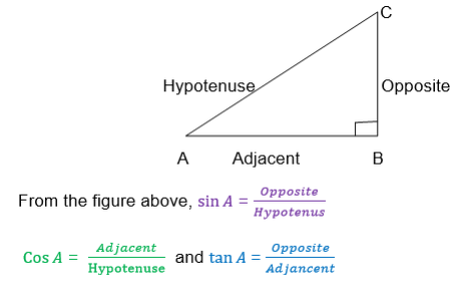Also we can define the above triangle ratios by using a unit Circle centered at the origin.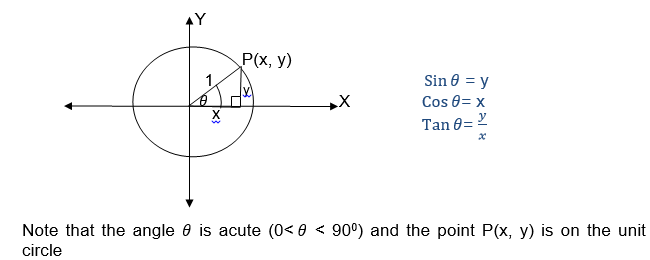If θis an obtuse angle (900<θ<1800) then the trigonometrical ratios are the same as the trigonometrical ratio of 1800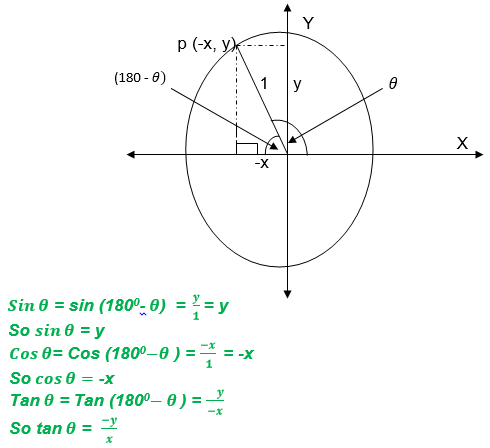The angles are as given below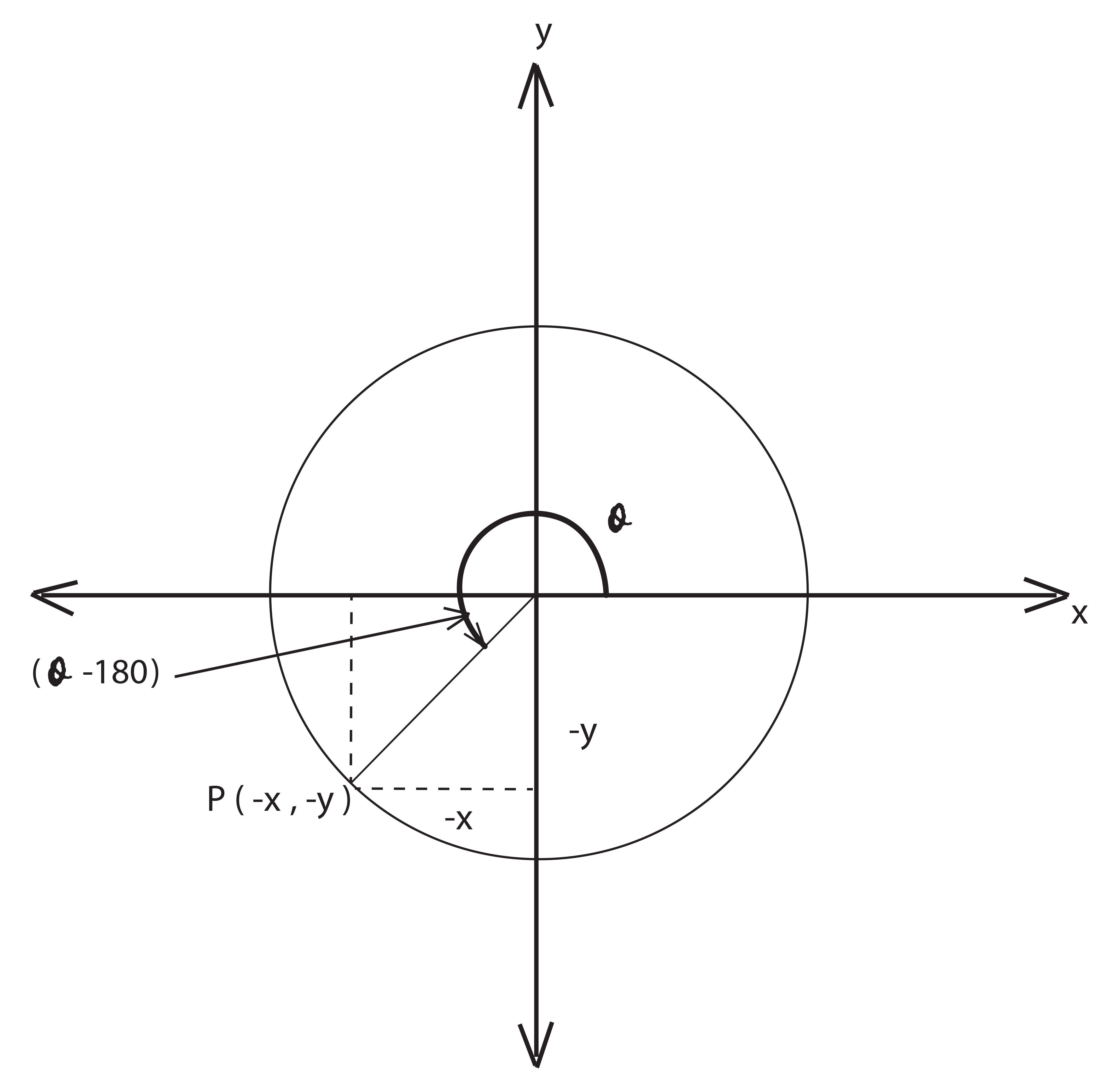If θ is reflex angle (180<θ<270) then the trigonometric ratios are the same as that of θ-180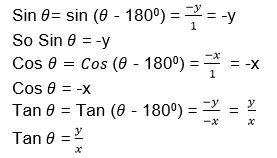If θis a reflex angle (2700< θ< 3600), then the trigonometrical ratios are the same as that of 360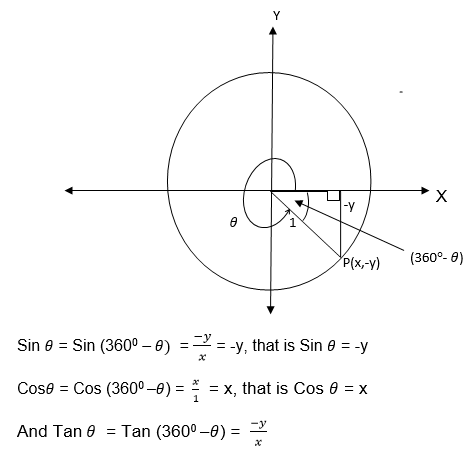We have seen that trigonometrical ratios are positive or negative depending on the size of the angle and the quadrant in which it is found.
The result can be summarized by using the following diagram.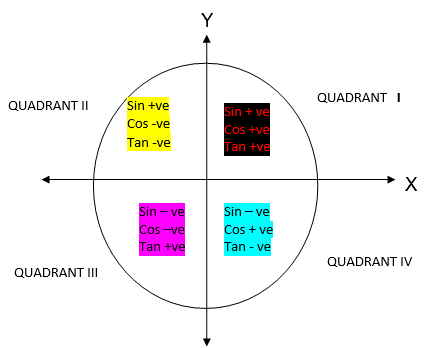Trigonometric Ratios to Solve Problems in Daily Life
Apply trigonometric ratios to solve problems in daily life
Example 1
Write the signs of the following ratios
1. Sin 1700
2. Cos 2400
3. Tan 3100
4. sin 300
Solution
a)Sin 1700
Since 1700 is in the second quadrant, then Sin 170= Sin (1800-1700) = Sin 100
∴Sin 1700 = Sin 100
b) Cos 2400 = -Cos (2400-1800)= -Cos 600
Therefore Cos 2400= -Cos 600
c) Tan 310= -Tan (3600-3100) = - Tan 500
Therefore Tan 3100= -Tan 500
d) Sin 3000= -sin (3600-3000) = -sin 600
Therefore sin 3000= - Sin 600
Relationship between Trigonometrical ratios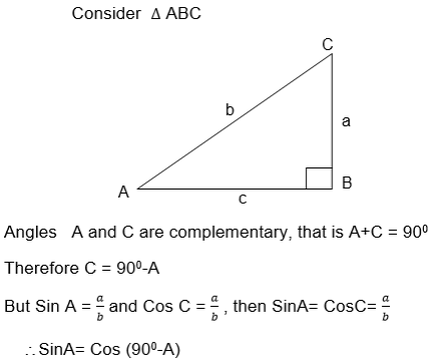The above relationship shows that the Sine of angle is equal to the cosine of its complement.
Also from the triangle ABC above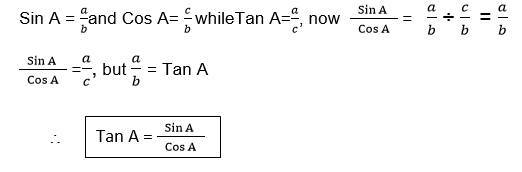Again using the ΔABC
b2 = a2+c2 (Pythagoras theorem)
And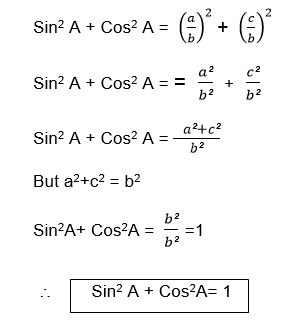Example 2
Given thatA is an acute angle and Cos A= 0.8, find
1. Sin A
2. tan A.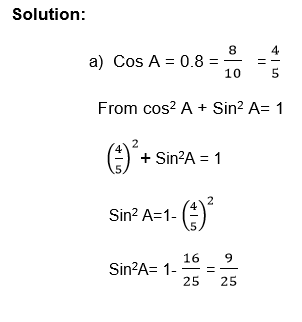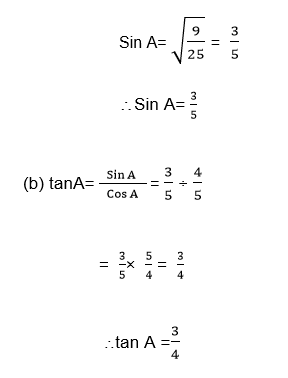Example 3
If A and B are complementary angles,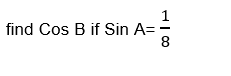Solution
If A and B are complementary angle
Then Sin A = Cos B and Sin B = Cos A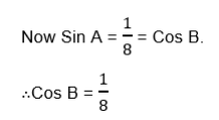Example 4
Given that θand βare acute angles such that θ+ β= 900 and Sinθ= 0.6, find tanβ
Solution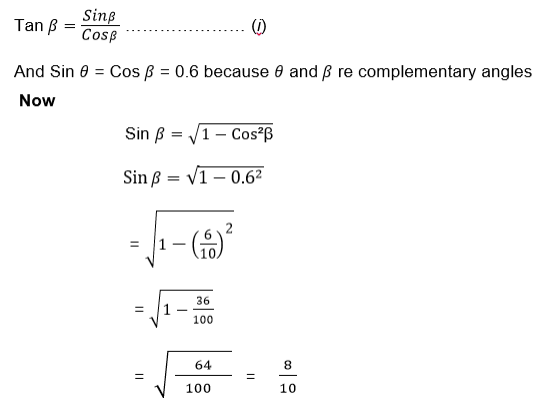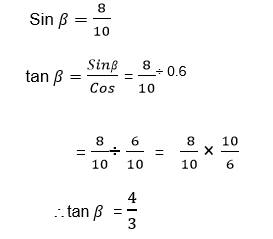Exercise 1
For practice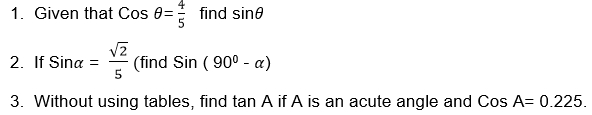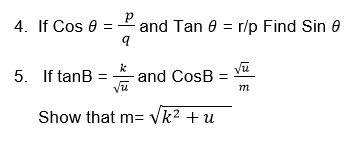Sines and Cosines of Angles 0 Such That -720°≤ᶿ≥ 720°
Find sines and cosines of angles 0 such that -720°≤ᶿ≥ 720°
Positive and Negative angles
An angle can be either positive or negative.
Definition:
Positive angle: is an angle measures in anticlockwise direction from the positive X- axis
Negative angle: is an angle measured in clockwise direction from the positive X-axis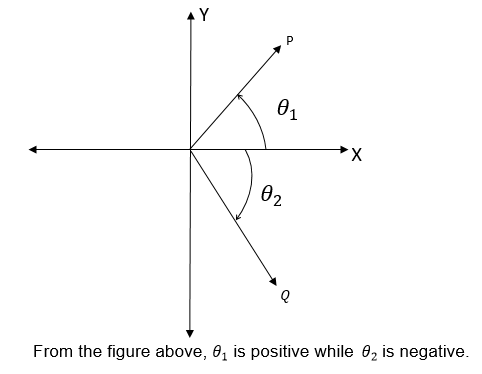Facts: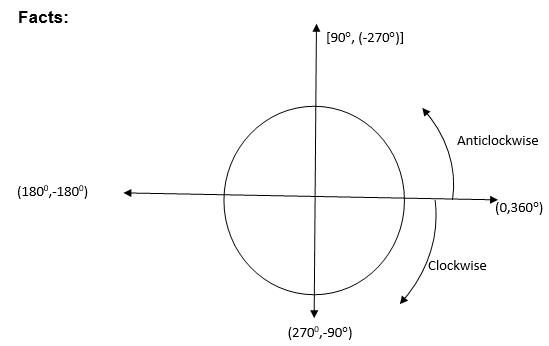1. From the above figure if is a positive angle then the corresponding negative angle to is (- 3600) or (+ - 3600)
2. .If is a negative angle, its corresponding positive angle is (360+)
Example 5
Find thecorresponding negative angle to the angle θif ;
1. θ= 580
2. θ= 2450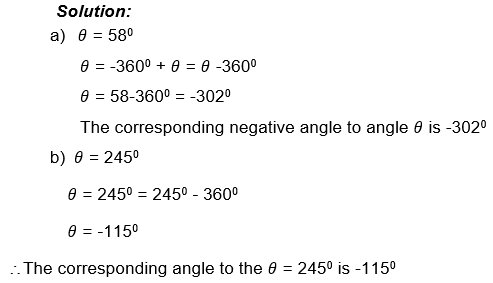Example 6
What is the positive angle corresponding to - 46°?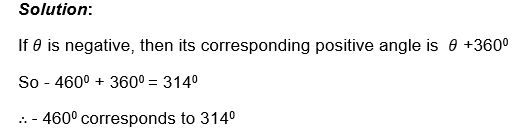SPECIAL ANGLES
The angles included in this group are 00, 300, 450, 600, 900, 1800, 2700, and 3600
Because the angle 00, 900, 1800, 2700, and 3600, lie on the axes then theirtrigonometrical ratios are summarized in the following table.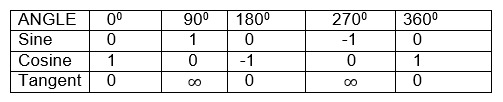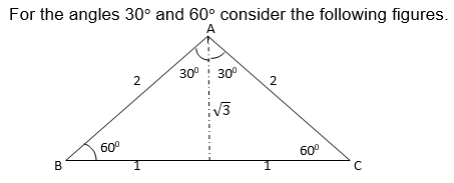The ∆ ABC is an equilateral triangle of side 2 units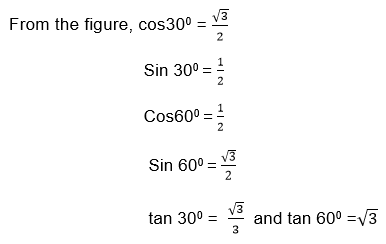For the angle 450 consider the following triangle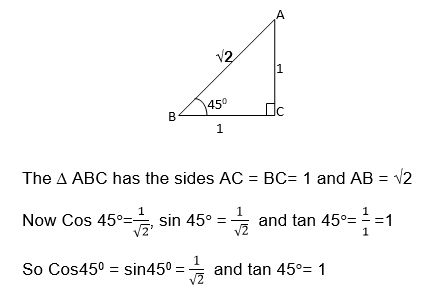The following table summarizes the Cosine, Sine, and tangent of the angle 30, 450 and 600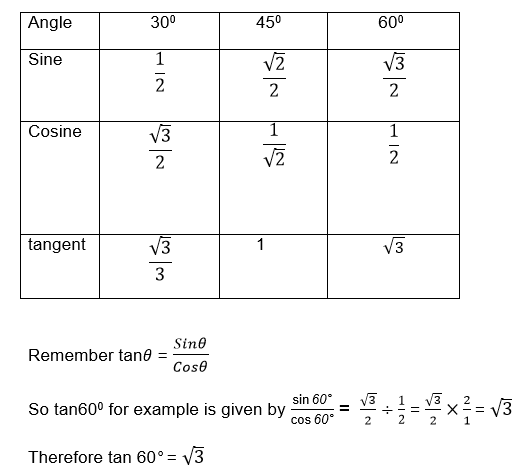NB: The following figure is helpful to remember the trigonometrical ratios of special angles from 0°to 90°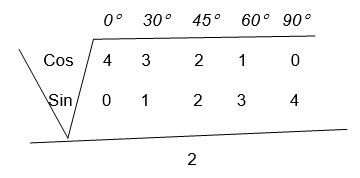If we need the sines of the above given angles for examples, we only need to take the square root of the number below the given angle and then the result is divided by 2.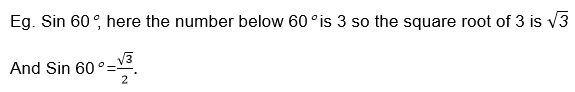Example 7
Find the sine,cosine and tangents of each of the following angles
1. -1350
2. 1200
3. 3300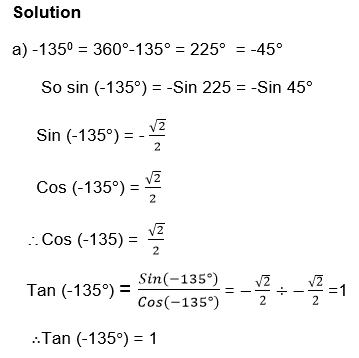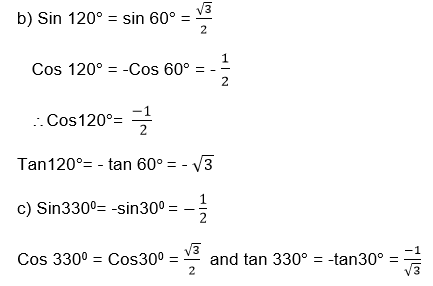Example 8
Find the value of θif Cos θ= -½ and θ≤ θ≤ 360°
Solution
Since Cos θis – (ve), then θlies in either the second or third quadrants,
Now - Cos (180 –θ= - Cos (θ+1800) = -½= -Cos600
So θ= 1800-600 = 1200 or θ= 1800 + 600 = 2400
θ= 1200 0r θ=2400
Example 9
Consider below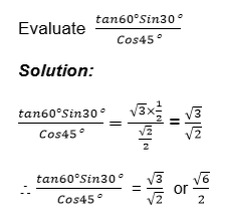Exercise 2
Solve the Following.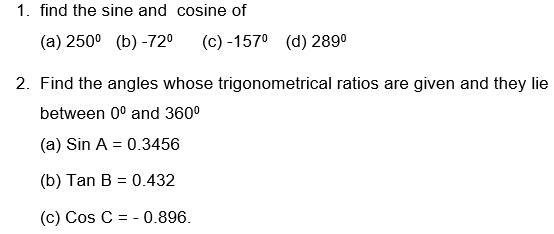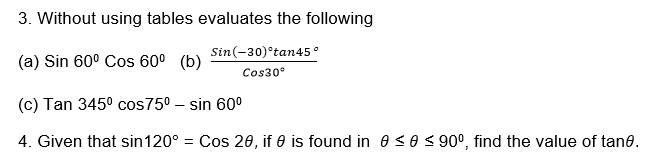The Graphs of Sine and Cosine
Draw the graphs of sine and cosine
Consider the following table of value for y=sinθ where θranges from - 360°to 360°For cosine consider the following table of values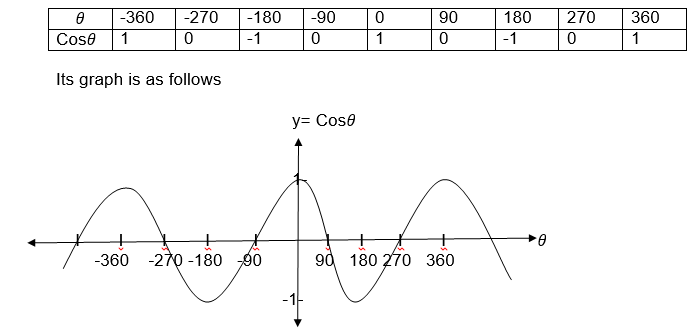From the graphs for the two functions a reader can notice that sinθand cosθboth lie in the interval -1 and 1 inclusively, that is -1≤sinθ1 and -1≤cosθ≤1 for all values of θ.
The graph of y= tanθis left for the reader as an exercise
NB: -∞≤ tanθ≤∞the symbol ∞means infinite
Also you can observe that both Sinθnd cosθrepeat themselves at the interval of
360°, which means sinθ= sin(θ+360) = sin(θ+2x3600) etc
and Cosθ=(Cosθ+3600)= Cos(θ+2x3600)
Each of these functions is called a period function with a period 3600
1. Usingtrigonometrical graphs in the interval -3600≤θ≤3600
Find θsuch that
1. Sin= 0.4
2. Cos= 0.9
solutionExample 10
Use the graph of sinθto find the value ofθif
4Sinθ= -1.8 and -3600 ≤θ≤3600
Solution
4Sinθ= -1.8
Sinθ= -1.8÷4 = -0.45
Sinθ= -0.45
So θ= -1530, -270, 2070, 3330
The graphs of sine and cosine functions
Interpret the graphs of sine and cosine functions
Example 11
Use thetrigonometrical function graphs for sine and cosine to find the value of
1. Sin (-400)
2. Cos (-400)
Solution
1. Sin (-400)= - 0.64
2. Cos (-400)= 0.76

The Sine and Cosine Rules
Derive the sine and cosine rules
Consider the triangle ABC drawn on a coordinate plane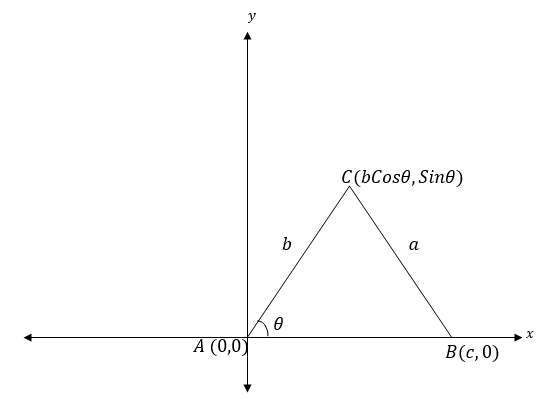From the figure above the coordinates of A, B and C are (0, 0), (c, 0) and(bCosθ, bSinθ) respectively.
Now by using the distance formula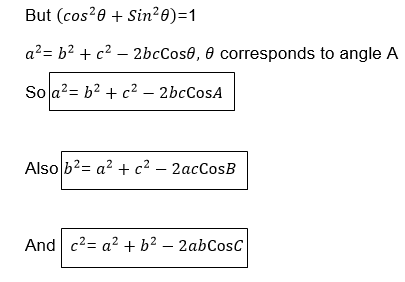SINE RULE
Consider the triangle ABC below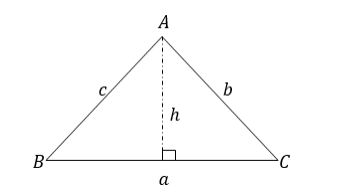From the figure above,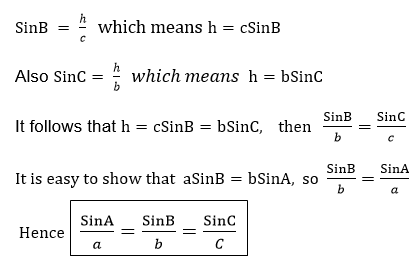Note that this rule can be started as “In any triangle the side are proportional to the Sines of the opposite angles”

The Sine and Cosine Rules in Solving Problems on Triangles
Apply the sine and cosine rules in solving problems on triangles
Example 12
Find the unknown side and angle in a triangle ABC given that
a= 7.5cm
c= 8.6cm and C= 80°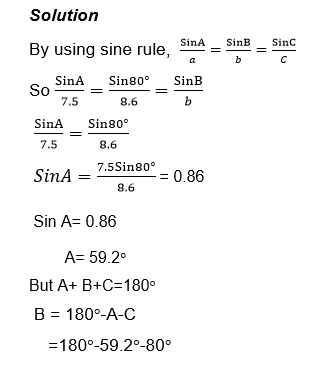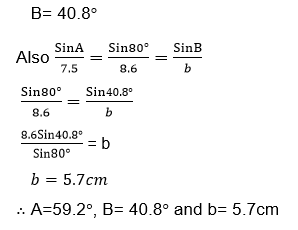Find the unknown sides and angle in a triangle ABC in which a= 22.2cmB= 86°and A= 26°
Solution
By sine rule
Sin A= sin B= Sin C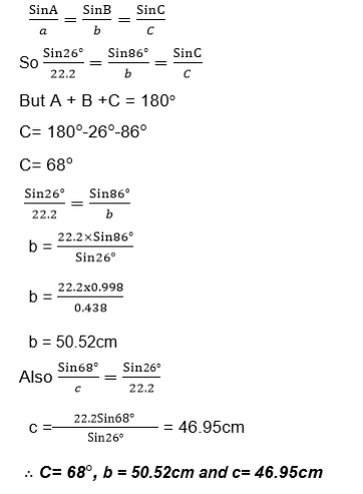Example 13
Find unknown sides and angles in triangle ABC
Where a=3cm, c= 4cm and B= 30°
Solution
By cosine rule,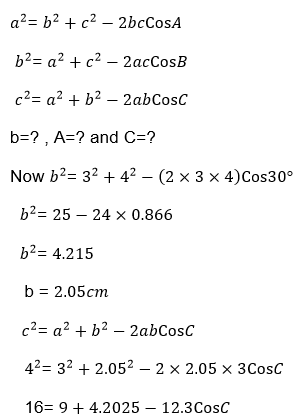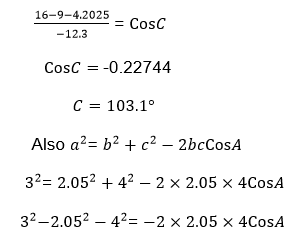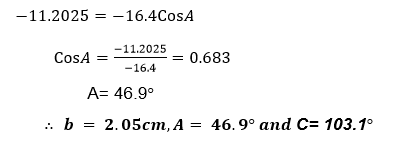Example 14
Find the unknown angles in the following triangle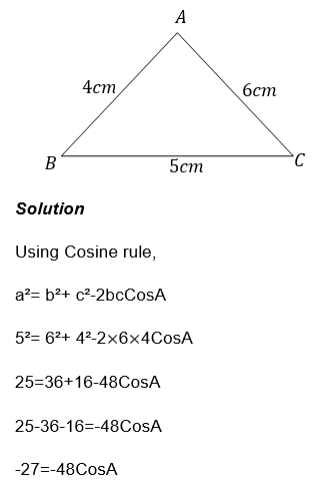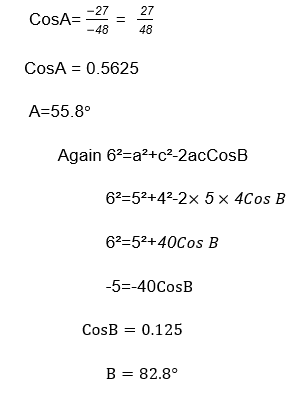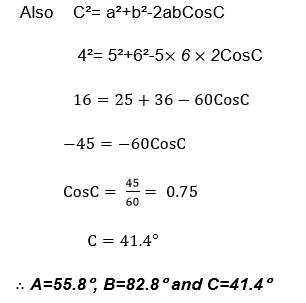Exercise 3
1. Given thata=11cm, b=14cm and c=21cm, Find the Largest angle of ΔABC
2. If ABCD is a parallelogram whose sides are 12cm and 16cm what is the length of the diagonal AC if angle B=119°?
3. A and B are two ports on a straight Coast line such that B is 53km east of A. A ship starting from A sails 40km to a point C in a direction E65°N. Find:
1. The distance a of the ship from B
2. The distance of the ship from the coast line.
4. Find the unknown angles and sides in the following triangle.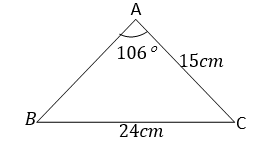5. <!--[endif]-->A rhombus has sides of length 16cm and one of its diagonals is 19cm long. Find the angles of the rhombus.

The Compound of Angle Formulae or Sine, Cosine and Tangent in Solving Trigonometric Problems
Apply the compound of angle formulae or sine, cosine and tangent in solving trigonometric problems
The aim is to express Sin (α±β) and Cos (α±β) in terms of Sinα, Sinβ, Cosαand Cosβ
Consider the following diagram: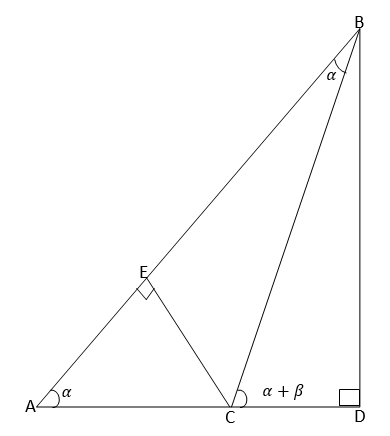From the figure above <BAD=αand <ABC=βthus<BCD=α+β
From ΔBCD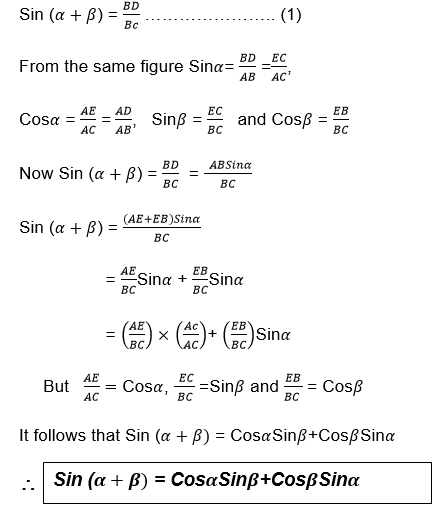For Cos(α±β) Consider the following unit circle with points P and Q on it such that OP,makes angleα with positive x-axis and OQ makes angle βwith positive x-axes.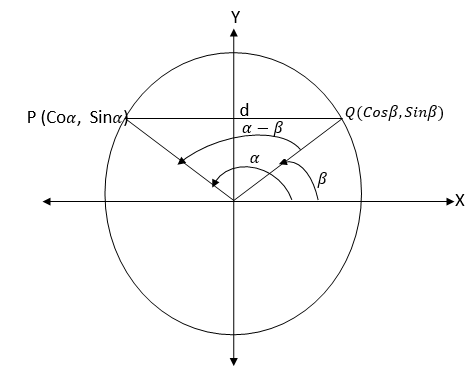From the figure above the distance d is given by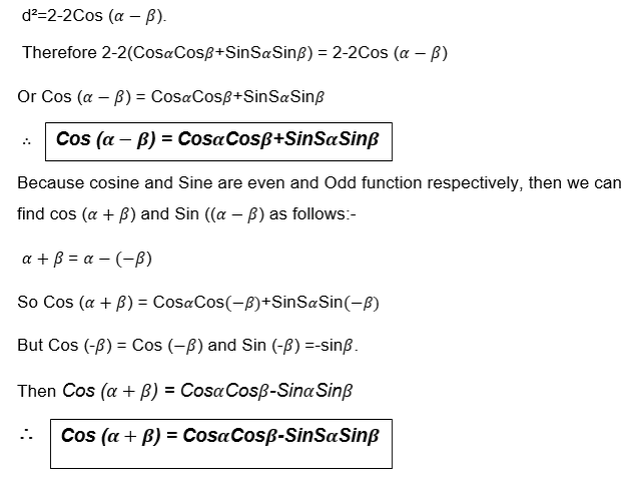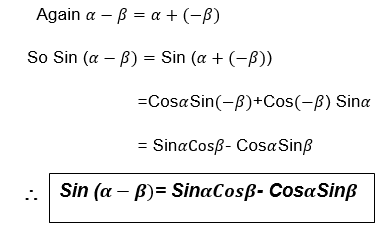In general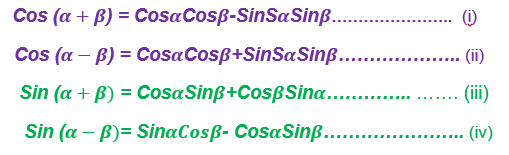Example 15
1. Withoutusing tables find the value of each of the following:
1. Sin 75°
2. Cos105
Solution: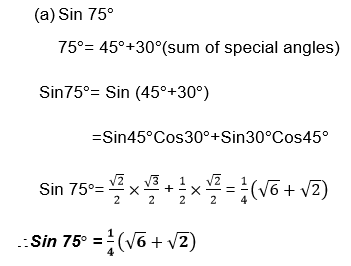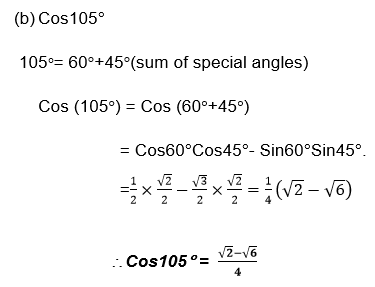Example 16
Find:
1. Sin150°
2. Cos 15°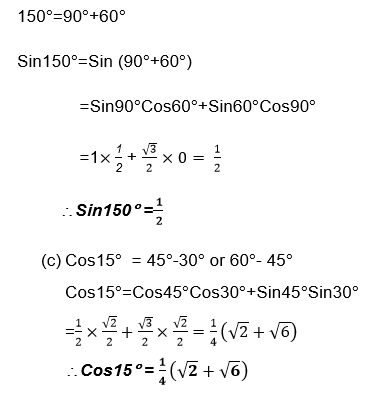Exercise 4
1. Withoutusing tables, find:
1. Sin15°
2. Cos 120°
2. FindSin 225° from (180°+45°)
3. <!--[endif]-->Verify that
1. Sin90° = 1 by using the fact that 90°=45°+45°
2. Cos90°=0 by using the fact that 90°=30°+60°
4. <!--[endif]-->Express each of the following in terms of sine, cosine and tangent of acute angles.
1. Sin107°
2. Cos300°
5. <!--[endif]-->By using the formula for Sin (A-B), show that Sin (90°-C)=Cos C